Subscribe to our newsletter to receive the latest news and events from TWI:

# Risk-Based Life Assessment of Corroding Pipelines

## Probabilistic Remnant Life Assessment of Corroding Pipelines within a Risk-Based Framework

Dr A Muhammed and Mr J B Speck

Paper presented at ASRANet International Colloquium 2002, 8 -10 July 2002, University of Glasgow, Scotland

## Abstract

This paper describes the application of a risk-based approach to the assessment of corroding offshore pipelines with the aim of optimising maintenance and replacement schedules. It describes an overall risk-based framework in which initial screening and risk ranking is followed by more detailed probabilistic assessment of the pipelines most at risk of failure. Initial screening is based on a semi-quantitative assessment of factors including pipeline inventory, historical and anticipated operating conditions, active damage mechanisms and potential failure consequences. Initial screening is formalised using TWI's RISKWISE TM risk assessment software, which deals with both likelihood and failure consequences for all pipelines. The RISKWISE TM output also includes a remaining life estimate based on conservative input parameters. Detailed probabilistic analysis is performed for the pipelines with low estimates of remaining life or high risk ranking, based on the results of the initial screening assessment. The probabilistic analysis considers both leakage and rupture of the pipelines, based on the modified ASME B31G. A major feature of the probabilistic model is the extrapolation of limited inspection data to cover longer pipeline lengths. Example calculations are presented based on a case study involving the assessment of about 260 subsea pipelines consisting of both water injection and oil production pipelines. The assessment results include both remaining life estimates and sensitivity studies. The method described provides a basis for determining maintenance and inspection priorities and ultimately the development of pipeline replacement strategies.

## Introduction

A method for the application of a risk-based approach to the assessment of corroding offshore pipelines is described. The approach involves an overall risk-based framework in which initial screening and risk ranking is followed by more detailed probabilistic assessment of the pipelines most at risk of failure. The study described was carried out with the aim of optimising pipeline maintenance and replacement schedules.

## Initial risk ranking

The study began with a review of all potential damage corrosion mechanisms for the pipelines. This produced a list of credible internal mechanisms including microbial corrosion, oxygen induced corrosion and CO 2 corrosion.

Firstly, a risk-ranking was produced using qualitative likelihood and consequence ratings in order to establish assessment priorities. Likelihood was based on factors such as process fluid corrosivity while consequence was assessed on the basis of the production criticality of the pipeline. This allowed very low risk pipelines to be eliminated from further consideration. Figure 1 shows a typical likelihood and consequence matrix from an initial risk ranking exercise.

A semi-quantitative assessment was carried out using TWI's RISKWISE TM assessment procedure for pipelines. This involved an estimation of the pipeline remaining life based on failure likelihood factors which were centered on current condition, likelihood of failure within specified forward time frames (e.g. 5, 10 and 15 years) and effectiveness of any inspections. These factors are used within the RISKWISE TM software to determine remaining life indicators (RLI). RLI is analogous to a remaining life estimate but it is termed an indicator because it is only intended to give a measure of the remaining life based on the change in the failure likelihood over time. Input data used in the RLI calculation are worst-case parameter estimates such that the RLI under-estimates remaining life compared to the more quantitative probabilistic method. In the case study described, pipelines with RLI of less than 5 years were selected as critical lines for the more detailed probabilistic assessment.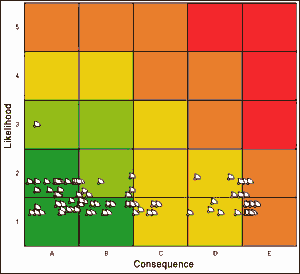Fig. 1. Estimates of risk

## Probabilistic corrosion assessment

### Analysis methods

The probabilistic assessment involved the formulation of a methodology for estimating the probability of failure by leakage or rupture, implementation in appropriate software and application to all critical pipelines. The work also involved analysis of input data to derive statistical distributions for various input parameters.

In general, failure probability methods use a number of techniques to estimate the probability of having combinations of uncertain variables that result in the occurrence of a failure event. Most practical problems can be thought of in terms of the interaction between a distribution of load effects and the resistance distribution as illustrated in Fig.2. In simple terms, all the analysis methods seek to establish the degree of overlap between the distributions. This depends essentially on the separation between the means of the distributions and the spread in each distribution. The area of overlap, and hence failure probability (P f), decreases with increasing separation between µ R and µ L while P f increases with increasing spread ( σ R or σ L) in either distribution.

In these analyses, probability distributions are used in preference to absolute values because the loading effects and resistance factors are subject to uncertainties that result from several sources. For example, for pipelines subject to internal pressure, uncertainties may arise from variability in material strength, pipe wall thickness and other geometrical properties, and fluctuations in internal pressure.

The discussion above involving two variables can easily be extended to cases involving several variables. For instance, a general expression for the failure condition or limit state may be stated as:

Z = G(X 1, X 2,.....X n)



where X 1, X 2,.....X n represent basic variables e.g. material yield strength, defect height, operating pressure, etc. and G is a valid mathematical expression defined such that failure occurs when Z is less than or equal to 0. This expression is known as the 'limit state equation'.

The required calculation for failure probability is: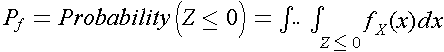where f X is the joint probability density function for the n-dimensional vector x of basic variables.

Several methods are available for the estimation of failure probability for a given uncertain event such as Eq.. These include Monte Carlo simulation, first order (FORM) and second order reliability (SORM) methods. The various methods for failure probability estimation have been widely published [1,2] and are implemented in commercial software products.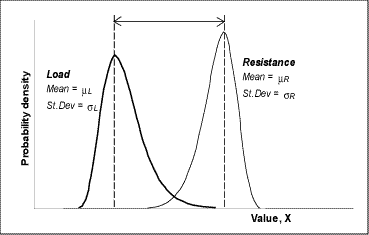Fig. 2. Illustration of failure probability analysis concept using the interaction between loading and resistance effects

Simulation methods such as Monte Carlo simulation involve generating random numbers for basic variables (X 1, X 2,..X n), at frequencies based on specified probability distributions for the variables, and checking whether failure is predicted or not (i.e., is Z<=0 ?) for each set of random sample values. This process is repeated several times and the ratio of the number of failures to the total number of simulations gives an estimate of the failure probability.

First order and second order reliability methods (FORM and SORM) use numerical procedures to simplify the joint probability density function f X in Eq. and sometimes to approximate the limit state equation, in the failure probability calculation. Unlike the simulation techniques, which may be time-consuming in low probability analysis, FORM and SORM are very fast in most practical cases.

In the present work, FORM and SORM were adopted as the primary method of estimating failure probabilities. Monte Carlo simulation was used to occasionally check the results.

## Probabilistic model

The probabilistic model was designed so that the failure event is defined as the occurrence of leakage or rupture at an internal corrosion defect in the pipeline. The failure model was defined in terms of depth of corrosion with the limit state equation written as:

Z = d c - d(t)



where d c is the critical corrosion depth for rupture or leakage and d(t) is the depth of corrosion at a given time t. In this formulation of the limit state equation, Z ≤0 constitutes failure while Z>0 is a safe condition. In the conceptual illustration of Fig.2, d c constitutes a resistance effect while d(t) is the loading effect.

The critical corrosion depth d c in Eq. was determined from the basic equations provided in the modified ASME B31G rupture criterion  for corroded pipes. The derivation of an expression for d c, begins with the modified B31G basic burst stress equation: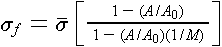where σ f is the hoop stress at failure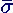is the flow stress, taken equal to yield strength + 10ksi (69MPa)

A is the corroded area in the longitudinal plane through the wall thickness

A 0 is LxB, mm 2

L is the axial extent of the corroded area, mm

B is the initial pipe wall thickness, mm

M is the 'Folias' factor, which is given by: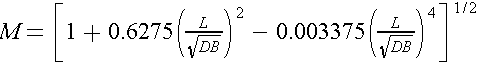; for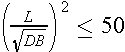and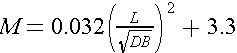; for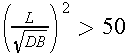where D is the diameter of the pipe, mm.

The corroded area, A in the above equation may be calculated in a number of ways. Where the data on corrosion profile is available, the area is computed fairly accurately. In the present work, only data on the maximum depth, d and the length of individual corrosion are known. In such cases, the modified B31G approach uses an approximate metal loss area, A, of 0.85 x d x L. Putting this approximation in Eq. and re-arranging the equation gives the maximum corrosion depth, d c, that may exist in the line for a failure stress corresponding to the specified (operating) hoop stress σ as: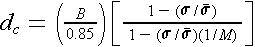From the form of the above equation, it was found that for very short corroded areas (i.e. small L) and at very low operating stresses, d c may be evaluated as being slightly greater than the wall thickness. Therefore, an upper bound value equal to the initial wall thickness, B was used in such cases. In the probabilistic analysis, the critical depth of corrosion was compared against the expected corrosion depth at different instances of time. The limit state equation was implemented in the form: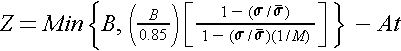where A is the corrosion rate in mm/year and t is the elapsed time in years.

The output from the probabilistic analysis based on Eq. for a given time t is the probability of failure (leakage or rupture) in the time interval from start-of-life to year t. The following equation is used to calculate the annual probability of failure at year t: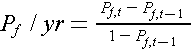Where P f,t is the probability of failure after 't' years in service. The denominator in the above equation allows for the fact that the pipeline, at the location in question survived up to year 't-1'

The failure probability calculations were carried out using the STRUctural RELiability software system (STRUREL) developed by RCP GmBH and licensed to TWI. STRUREL  is a commercial software within which failure conditions such as Eq. can be defined and probabilistic analysis conducted.

The results of the probabilistic analysis were checked for accuracy by running a selection of independent deterministic calculations to confirm that the FORM and SORM solutions satisfied the failure equation or limit state. This confirmed that the basic failure equations had been implemented properly.

## Case study - input data and statistical analysis

Example calculations are now presented based largely on a case study that involved the assessment of about 260 subsea pipelines. This consisted of water injection and oil production pipelines spread over two oil producing fields. A summary of the input data used in the probabilistic corrosion assessment is given in Table 1 and further details on the input assumptions are given below.

Table 1 General input data used for the probabilistic analyses

VariableDistributionMeanCOV, %
Initial wall thickness, B Normal 1.02 x nominal 4
Outside Diameter, D Fixed Nominal 0
Yield strength Normal 1.09 x SMYS 4
Operating stress, σ Fixed pD/2B, where p = pressure 0
Predictive model ratio, R* Normal 0.622 22.2
Corrosion area (mm/yr)† Extreme value 0.14 35.7
Corrosion defect length, L Fixed 450mm 0

Notes
COV = standard deviation divided by mean
* R is the ratio of the modified ASME B31G predicted failure stress to actual failure stress in pipe burst tests  (Mean = 0.622, Standard deviation = 0.138, COV = 22.18%)
† Baseline distribution before extrapolation quoted in the table (Extrapolated mean for a 3km long pipeline = 0.23 mm/year)

### Basic input data

Input data such as pipe geometrical properties, yield strength and operating stresses were readily derived from the design information available from the pipeline database. Pipe diameter and operating stress were considered as fixed values while some uncertainties were assumed on pipe wall thickness and yield strength values. In both cases, normal distributions were assumed with mean values related to nominal wall thickness and specified minimum yield strength (SMYS). This treatment is in-line with published work on probabilistic assessment. [5,6]

### Uncertainty in burst prediction model

The modified B31G predictive model for pipe rupture or leakage is designed to be conservative. Statistical information is provided on the relationship between the predicted failure stress (given by Eq.) and actual failure stresses obtained from pipe burst tests in the modified ASME B31G document  . The distribution of predicted to actual failure stress ratio, R, in the tests was found to be a normal distribution with a mean value of 0.622 and a standard deviation of 0.138. This suggests that on average, the actual failure pressure or stress is about 60% higher than the predicted value. This ratio was introduced into the probabilistic model by taking the stress σ in the limit state Eq. as operating stress multiplied by stress ratio R.

### Corrosion defect length

In this study, lengths reported from inspection were fitted to standard statistical distributions. The best fit obtained from the analysis was a lognormal distribution. However, a significant amount of variability was observed. Such large variability, particularly for a lognormal distribution, can lead to numerical problems in the probabilistic calculation routines. It was also not clear if the length of the corroded area would increase with time, it was therefore considered appropriate to use a conservative fixed value based on the distribution obtained. The equivalent mean + 2 standard deviations for the lognormal distribution, (widely accepted as a conservative estimate of a variable value) was about 450mm. This value was therefore assumed in the probabilistic analysis of pipelines. It was considered conservative because examination of a section of a failed water injection pipeline revealed that the corroded area that caused failure measured approximately 350mm in length. Therefore the assumed length of 450mm is conservative with respect to that known to have caused failure.

### Corrosion rate distribution

General

Corrosion rate distributions were mainly derived from available inspection data on the basis of the type of service. For example, inspection data on all water injection pipelines were processed to determine a basic corrosion rate distribution. Similarly, inspection data for oil production lines were combined to derive the appropriate corrosion rate distributions. The approach of combining data from several pipelines was necessary because inspection data was limited both in terms of the overall number of the pipelines inspected and the extent of inspection on individual pipelines. Typically, ultrasonic cable-operated inspection equipment introduced from one or both ends would inspect 500m to 800m end sections of a pipeline. This only provided a sample inspection as the pipelines were sometimes up to 7km long. To account for the limited nature of the inspections, extreme value distributions were fitted to the measured data and then extrapolations were carried out to estimate the maximum corrosion rates that might have been expected had the entire pipelines been inspected. In general, corrosion rate statistics were derived by dividing the metal loss reported from the inspection by the number of service years since commissioning.

Corrosion rate extrapolation for pipeline length

The method of extrapolation based on sample inspection has been used previously by a number of investigators. [7,8] The technique has been more comprehensively developed in a recent TWI work  in which several aspects of the methodology were investigated. In the present study, the maximum corrosion depths for approximately the same unit lengths of pipeline were fitted to an extreme value distribution using commercial statistical software. This gave a Type I extreme value distribution of maximum rates, which has the form: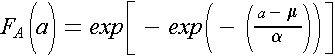where F A(a) is the probability of the corrosion rate A having a value less than 'a', µ is referred to as the location parameter and α is the scale parameter. In simple terms, the location parameter is the most likely corrosion rate and the scale parameter represents the extent of scatter of rates about the location parameter and therefore controls the shape of the distribution.

Extrapolation of the basic distribution to allow for potentially higher corrosion rates in uninspected regions of the lines is done by shifting the location parameter to a higher value. The location parameter of the shifted distribution is given by: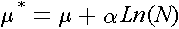where µ and α are the parameters of the basic distribution and N is the ratio of total pipeline length to the unit length from which worst data was extracted to derive the basic distribution. The above equation follows from extreme value theory and is quoted in several standard texts (e.g. see Ref  ). The extrapolation of Eq. is based on two fundamental assumptions: firstly, that the uninspected region is nominally similar to the region sampled and secondly, that the unit sections considered in extracting maxima are independent or at least that correlation is negligible. Therefore, the unit length of pipeline used was selected based on previous experience  to ensure independence. The other requirement of the sample being representative of the uninspected pipeline regions was particularly important in the present study as inspections were mostly limited to pipeline ends. However, a limited number of checks showed that variability in wall thickness loss stabilised within the typical 500m end lengths covered by the inspections. For example, see plot of wall thickness versus distance from line end in Fig.3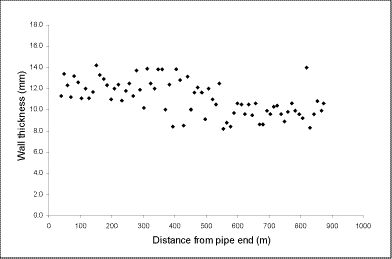Fig. 3. Typical wall thickness variation with distance from pipe end

A typical example of the basic extreme value distribution and the corresponding extrapolations for different pipeline lengths are shown in Fig.4. The effect of extrapolation is clearly seen as the entire distribution is shifted to higher corrosion rates. It should be noted that the shift is logarithmic with pipe length.

Validation against failure data

Leaks had been reported for a few of the water injection lines assessed in this exercise. The corrosion rates implied by these leaks were compared against the corrosion rate distributions obtained for the failed lines. These checks provided some validation for the procedure for determining corrosion rate distributions, as the rate implied by the leaks fell in the upper tail region of the distributions (e.g. see Fig.4). The two rates shown in Fig.4 are the minimum and maximum values from several failures. It is also noted from Fig.4 that the rates implied by the failures would be much less credible if basic corrosion rate distributions were not extrapolated for the length of the lines.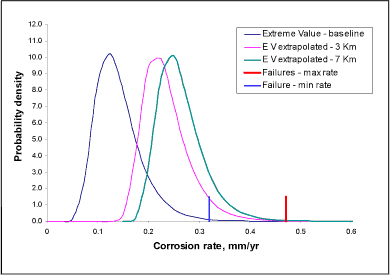Fig. 4. Extrapolation of corrosion rate distribution and comparison with rates implied by failures (full pipeline length 7Km)

## Case study - results of failure probability calculations

General

The results of the assessment are presented in the form of plots of annual failure probability against time. A typical plot is shown in Fig.5. The plot shows an initial assessment and an updated assessment following inspection of the individual pipeline. Updating is further discussed within the section describing the sensitivity study in this paper. For a plot such as that shown in Fig.5 to be used in determining a remaining life, a cut-off failure probability for the end of useful life has to be defined. This is often referred to as the target failure probability.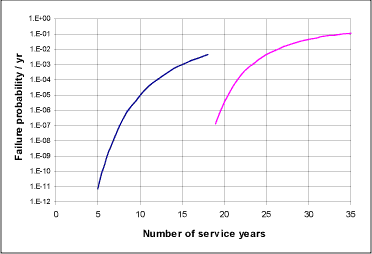Fig. 5.Annual failure probability - time plot and updating with inspection data

### Target failure probability and calibration with failure data

Two basic factors were considered in setting the target annual failure probability levels for determining the end of life and hence the pipeline remaining lives. Firstly, the target values recommended in offshore codes, standards and other published documents [10-12] were reviewed. In most cases these are based on safety classification, which for pipelines, is mainly related to the fluids transported and the pipeline location. Typically, three safety classes of 'low', 'normal' and 'high' are defined. In line with guidance in the offshore standards, [10,11] water injection lines may be categorised into 'low' safety class while oil production and gas lines may be considered 'high' safety class. The target failure probabilities recommended for design against a failure condition such as rupture (due to corrosion) in the standards and other relevant publications are summarised in Table 2. A second consideration in setting the target failure probability involved calibrating the results of the probabilistic assessment against the field experience in terms of reported leaks and failures in water lines. Analyses were conducted to estimate the annual failure probabilities for the years leaks were reported in these lines. This gave values in the range of 2.2x10 -3 to 7x 10 -2 for the lines. These failure probability values are broadly in line with the range of 10 -3 to 10 -2 per year recommended in the standards (see Table 2).

Table 2 Recommended annual target failure probabilities

LowNormalHigh
RP-F101
(DNV 1999)
10 -3 10 -4 10 -5
OS-F101
(DNV 2000)
10 -3 10 -4 10 -5
Sotberg et al (1997)
(SUPERB project)
10 -2 - 10 -3 10 -3 - 10 -4 10 -4 - 10 -5

Based on the two considerations outlined above, annual failure probabilities of 10 -3 to 10 -2 and 10 -5 were adopted for water injection and oil production lines respectively. The results of the probabilistic analysis for the water injection lines that failed in one field were closer to 10 -3, so this lower target was applied to all water lines in that field. Similarly, the higher target value of 10 -2 matched the results for water line failures in the second field, so the higher value was adopted.

### Sensitivity analyses

The sensitivity of the results of a probabilistic analysis to the various input parameters were evaluated by examining the so-called sensitivity factors produced from a FORM or SORM analysis. Figure 6 shows, in a pie chart, the sensitivity of results to input data for a typical water injection line. This shows clearly that corrosion rate is by far the most influential variable. Initial wall thickness and the burst failure prediction error, R, also have some effect. Of these variables, there is reasonable confidence in the estimates of initial wall thickness and on the prediction error R, while the appropriateness of the assumed corrosion rate distribution for individual pipelines is less certain particularly where such lines had not been inspected. The effect of inspection on corrosion rate distributions and consequently on predicted remaining life was explored through further sensitivity analyses.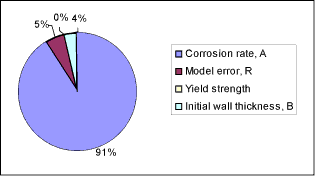Fig. 6. Sensitivity of analyses results to input vari ables for a typical water injection line

Firstly, it was noted that the limit state Eq. employed in the probabilistic model simply accumulates the metal loss from start of life and predicts failure when this is sufficient to cause a leak or rupture. If there is a high degree of uncertainty regarding the corrosion rate distribution, then the amount of metal loss assumed by the model after a given service period may be in significant error. Therefore for lines with in-service inspection, a second set of analyses was conducted utilising a different limit state equation based on the actual measured wall thickness distribution at the time of inspection. The time frame for this second analysis starts from the inspection date and essentially corrects the extent of metal loss assumed in the model to that observed from inspection. Figure 5shows failure probability plots for an initial assessment and the corresponding modified plot after updating with thickness measurements for the specific pipeline. The drop in failure probability estimate at the inspection time is a measure of the correction due to the availability of the inspection data on the individual pipeline. Due to the conservatism in the general analysis prior to updating for individual pipelines, the remaining life estimates in all cases considered increased as a result of the updating. The results of initial and updated analysis for 5 pipelines are given in Table 3, which show improvements in remnant life estimates of between 6 and 13 years.

Table 3 Effect of line-specific NDT data on remaining life estimate

PipelineLife estimate - no line specific NDT (years)Life estimate - line specific NDT used (years)Difference (years)
Line 1 2.3 18.3 16
Line 2 0.1 13.1 13
Line 3 9.5 15.5 6
Line 4 7.3 20.3 13
Line 5 2.7 12.7 10
Average     11.6

## Discussion

### Initial risk ranking

Initial screening produced results that were used to exclude low risk pipelines from further consideration while those with low remaining life estimates (less than 5 years) were identified for detailed probabilistic assessment. This provided a means of rapidly screening the pipelines making for economy of effort. The semi-quantitative RISKWISE TM assessment procedure as applied to the pipelines is intended to be a conservative screening level for the probabilistic assessments that follow.

### Probabilistic assessment

The corrosion rate distributions were validated against actual field failures and the end of life target failure probabilities were calibrated against reported failures, so it would be expected that the remaining life estimates are conservative. However, the sensitivity study showed that the calculated failure probability, and hence the remaining life estimates are very sensitive to the corrosion rate distribution assumed. The analyses showed that the corrosion rates derived from the combined inspection data, may underestimate the remaining life by up to 16 years, if thickness data specific to individual lines were not considered. This implies that the results may be overly conservative for some of the lines that had not been inspected. Nevertheless, the probabilistic analyses method provides remaining life estimates that can be used to determine maintenance and inspection priority as well as a basis for formulating a pipeline replacement strategy.

## Conclusions

A method is presented for the remnant life assessment of corroding pipelines. The method entails an initial risk ranking to identify the pipelines at high risk of failure. Detailed probabilistic remaining life assessments are then carried out on the identified pipelines. The probabilistic model allows for uncertainty in variables including material strength properties, corrosion area sizes and corrosion rates. Inspection data plays a major role in deriving corrosion rate distributions, and a major feature of the probabilistic model is the extrapolation of limited inspection data to cover longer pipeline lengths. Failure probability estimates are obtained from First Order Reliability Method (FORM) and verified by Monte Carlo simulations.

The results presented in this paper are largely based on a case study that involved the assessment of about 260 subsea pipelines consisting of both water injection and oil production pipelines. The assessment results included both remaining life estimates and sensitivity studies. The failure probability estimates were validated against reported pipeline failures in order to define the appropriate end-of-life target failure probabilities. The case study results provided a means of identifying the lines at the highest risk of failure. The sensitivity studies showed the potential benefit of conducting further inspections to provide improved corrosion rate estimates, and to better establish the condition of specific pipelines.

The method described provides a basis for determining pipeline inspection priority and ultimately for developing a replacement strategy.

## References

1. Ang A H-S and Tang W H: 'Probability concepts in engineering planning and design', Vol.II, Decision, Risk and Reliability, John Wiley, New York, 1984.
2. Melchers R E: 'Structural analysis and prediction'. Second edition, John Wiley & Sons, 1999.
3. Kiefner J F and Vieth P H: 'A modified criterion for evaluating the remaining strength of corroded pipe'. Project PR 3-805, AGA, December 1989.
4. RCP GmBH: 'STUREL - A structural reliability analysis program-system', Users Manual, RCP Consult, 1997.
5. Zimmerman I J E, Hopkins P and Sanderson N: 'Can limit spate design be used to design a pipeline above 80% SYMS?', 17 th International Conference on Offshore Mechanics and Arctic Engineering, OMAE 98-0902 ASME, 1998.
6. Jiao G et al: 'The SUPERB project: linepipe statistical properties and implications in design of offshore pipelines'. 1997 OMAE, Vol.V, Pipeline Technology, ASME 1997.
7. Buxton D C, Cottis R A and Scarf P A: 'Life prediction in corrosion fatigue' in Parkins R N (Ed), Life Prediction of Corrodible Structures, Vol.II, 1273-1282, NACE, 1994, ISBN 1-877914-60-6.
8. Laycock P J, Cottis R A and Scarf P A: 'Extrapolation of extreme pit depths in space and time'. J Electrochen, Soc., Vol.137, No.1, January 1990.
9. Schneider C R A, Muhammed A and Sanderson R M: 'Predicting the remaining lifetime of in-service pipelines based on sample inspection data'. Journal of the British Institute of Non-Destructive Testing,Insight, Vol.43, No.2, February 2001.
10. RP-F101: 'Corroded pipelines'. Recommended Practice, RP-F101, DNV, 1999.
11. OS-F101:'Offshore Standard- Submarine Pipeline Systems' DNV 2000.
12. Sotberg T et al: 'The SUPERB Project: Recommended target safety levels for limit state based design of offshore pipelines' Conf. Offshore Mechanics and Arctic Engineering (OMAE), Vol. V-pipelinetechnology, ASME, 1997